Students can Download Maths Chapter 1 Numbers Additional Questions and Answers, Notes Pdf, Samacheer Kalvi 8th Maths Book Solutions Guide Pdf helps you to revise the complete Tamilnadu State Board New Syllabus and score more marks in your examinations.

Tamilnadu Samacheer Kalvi 8th Maths Solutions Term 3 Chapter 1 Numbers Additional Questions

Question 1.
Find the least number by which 1100 must be multiplied so that the product becomes a perfect square. Also, in each case find the square root of the perfect square so obtained.
Solution:
We find 1100 = 2 × 2 × 5 × 5 × 11 =2² × 5² × 11
∴ The prime factor 11 has no pair.
∴ If we multiply 1100 by 11, then the product becomes a perfect square.
∴ New number = 1100 × 11 = 12100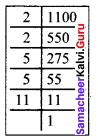12100 = 2² × 5² × 11²
$$\sqrt{12100}$$ = 2 × 5 × 11 = 110
Square root of the new number = 110Question 2.
Find the square of 509 using (a + b)² = a² + 2ab + b²
Solution:
509² = (500 + 9)² = 500² + 2 x 500 x 9 + 9²
= 250000 + 9000 + 81
509² = 259081

Question 3.
1 + 3 + 5 + 7 + 9 + 11 + 13 + 15 + 17 + 19 + 21 + 23
Solution:
Given sum is the sum of first 12 odd natural numbers.
Sum of first n odd natural numbers is n².
∴ 1 +3 + 5 + 7 + 9 + 11 + 13 + 15 + 17 + 19 + 21 + 23 = 12² = 144

Question 4.
Write a Pythagorean triplet whose one number is 110
Solution:
Here let 2m = 110
m = 55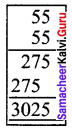m² – 1 = 55² – 1 = 3025 – 1 = 3024
m² + 1 = 55² + 1 = 3025 + 1 = 3026
∴ Pythagorean triplet is 110, 3024, 3026.

Question 5.
Find the square root of 10 $$\frac{2}{3}$$ correct to three places of decimal.
Solution:
10 $$\frac{2}{3}$$ = 10.6666…….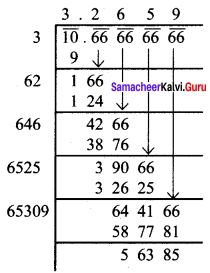$$\sqrt{10 \frac{2}{3}}=3.2659 \Rightarrow \sqrt{10 \frac{2}{3}}$$ = 3.266 correct to three places of decimal.

Question 6.
Find the square root of 0.053361
Solution: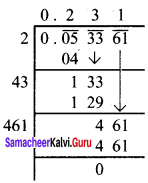$$\sqrt{0.053361}$$ = 0.231Question 7.
Three numbers are in the ratio 2:3:4. The sum of their cubes is 33957. Find the numbers.
Solution:
Let the numbers be 2x, 3x and 4x
(2x)³ + (3x)³ + (4x)³ = 33957
8x³ + 27x³ + 64x³ = 33957
99x³ = 33957
x³ = $$\frac{33957}{99}$$
x³ = 343
x³ = 7 × 7 × 7
x³ = 7³
x = 7
∴ The numbers are 2x = 2 × 7 = 14
3x = 3 × 7 = 21
4x = 4 × 7 = 28

Question 8.
The volume of a cube is 9261000 m³. Find the side of the cube?
Solution:
Volume of the cube = side x side x side
side x side x side = 9261000
side = $$\sqrt{9261×1000}$$ = $$\sqrt{9261}$$ × $$\sqrt{1000}$$
= $$\sqrt{3^{3}×7^{3}}$$ × $$\sqrt{10×10×10}$$ = 3 × 7 × 10 = 210
∴ Side of the cube = 210 m

Question 9.
If the diameters of the sun and Earth are 1.4 × 109 m and 1.275 × 107 m respectively. compare these two.
Solution: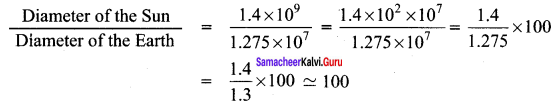So the diameter of the Sun is about 100 times the diameter of the Earth.

Question 10.
The size of a red blood cell is 0.000007 m and that of a plant, cell is 0.00001275 m. Compare these two.
Solution: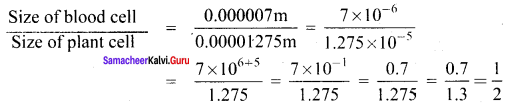∴ RBC size if half the size of a plant cell.